# Get Matrix between Frames in Components

Hello,

i cannot get the correct result with following problem. The two matrix (green and orange) I calculated rigth and controlled it with measuring! I cant find the right way to calculate the matrix between the two frames (one is the base and the other can be somewhere).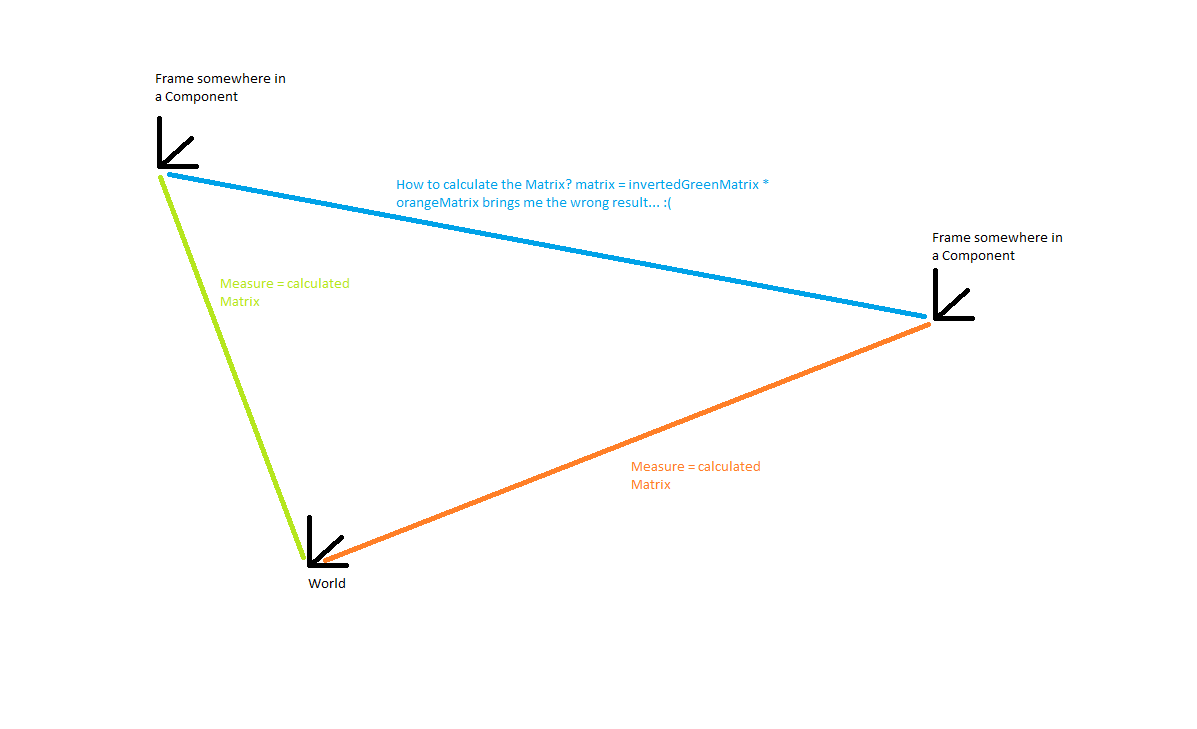I try the calculation like the example in Help, but get the wrong result, controlled by measure…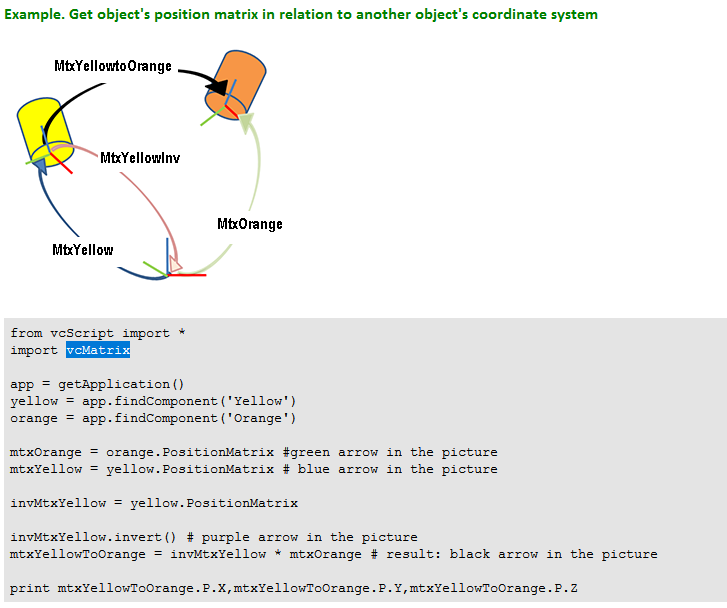Thank you & Best Regards

Feature

When you took the measurement, what was the coordinate system selected in the Measure tool task pane: World, Parent, or Object?

If you multiple the inverse of green * green… what do you think the measurement would be? If you are transforming orange to a different space, for example green, what do you think the measurement would be in parent coordinate system from green to orange?

Hy zesty,

In my case I think Measure with World and Object should bring the same correct measure but I prefer Object!

Maybe my result was already correct, I simplified my Testexample and remove some rotations of my TargetFrames, then it lookes nice. Maybe my tests was to difficult for me to understand because I cannot check it by measure! Look at my example: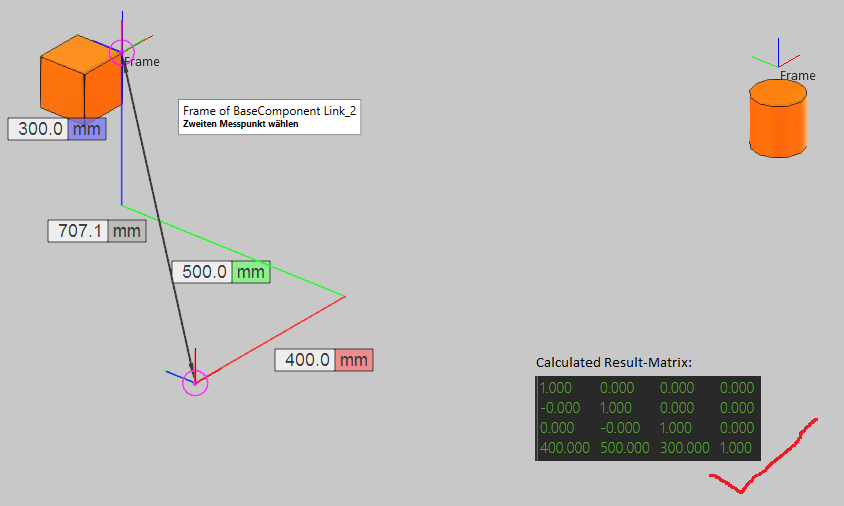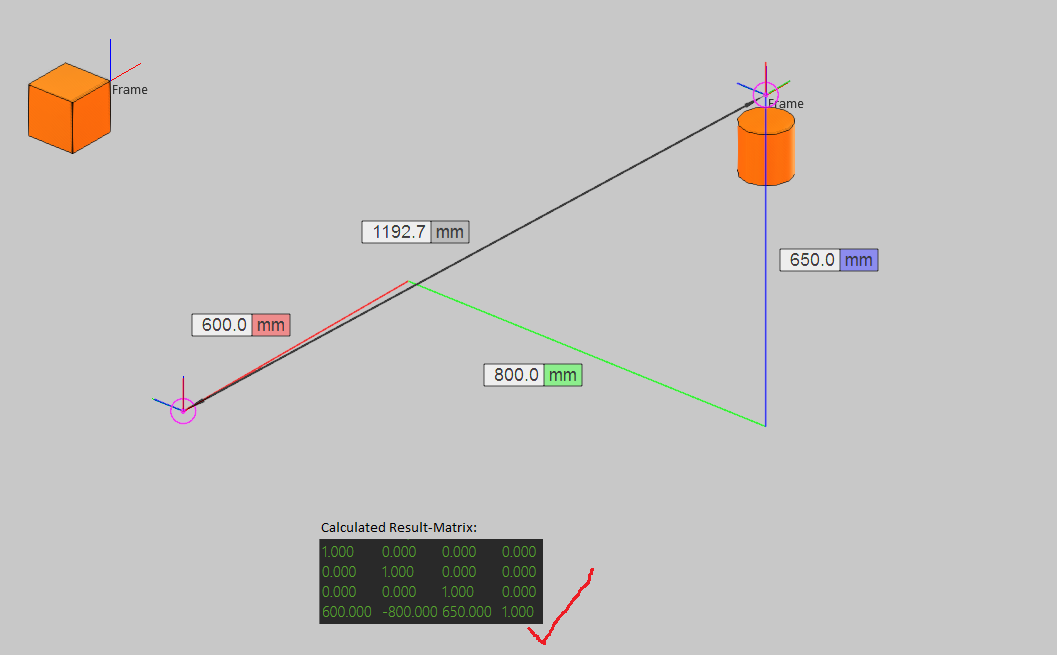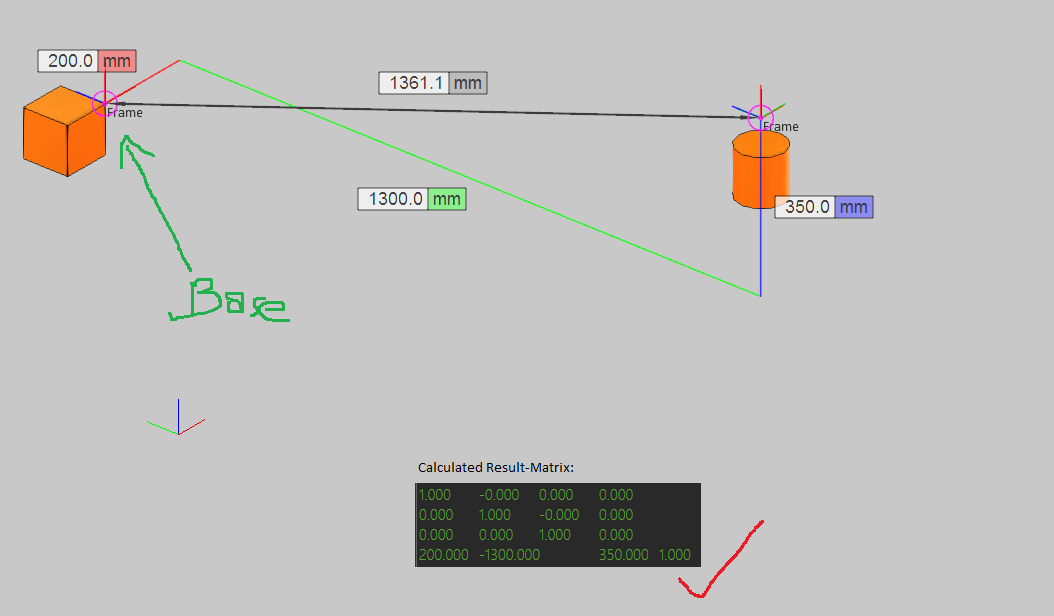So my question is how to interprete the result Matrix if the two Frames have some rotations. -> What I was expect to find was a Vector in the Matrix that I can control by the same measure like in the last picture! -> Look at the result in the next picture, how can I interprete this? (Could this be right)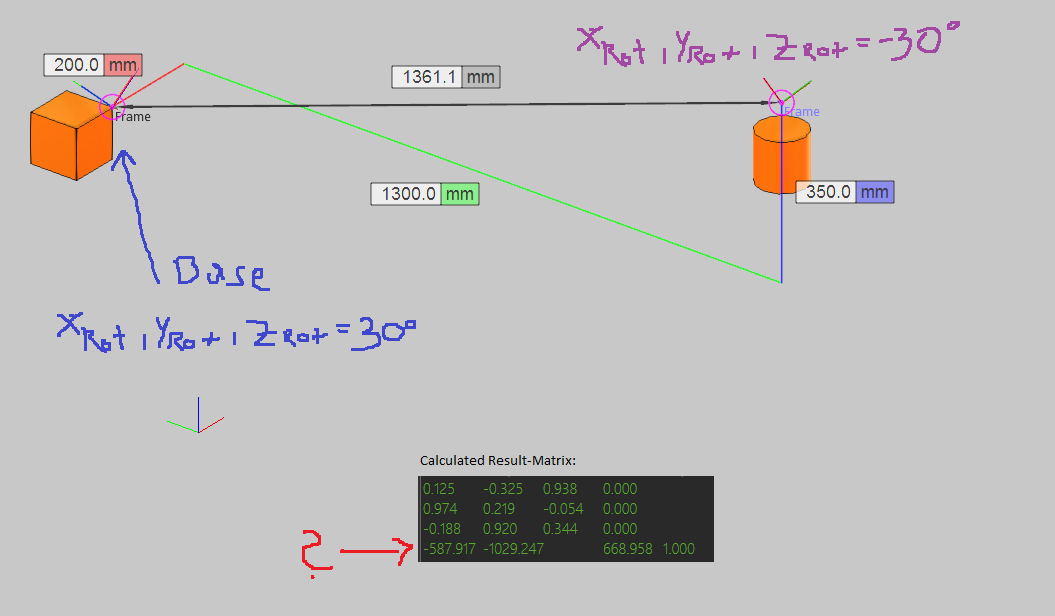Thank you & Best Regards

Feature

The measure tool should allow you to measure distance and angle. Perhaps a lesson in Academy is needed on this topic. Perhaps lesson 11 of modeling a mobile robot can help with showing how to account for angle offsets, but I will see what I can do tomorrow to make an example for you. If others have time now, please share.

The measure tool should allow you to measure distance and angle. Perhaps a lesson in Academy is needed on this topic.
Is possible! ;)
Perhaps lesson 11 of modeling a mobile robot can help with showing how to account for angle offsets, but I will see what I can do tomorrow to make an example for you.
An example would be very helpfull for me! :)

Thank you & Best Regards

Feature

Sorry, I am not able to make an example. Others will have to help.

Hi,

When converting matrix form world coordinates into some other coordinate system the rotation of the new reference system will effect the position values in the matrix. Consider the image below. If you calculate transformation matrix from Base to Frame you will have position values displayed in the image because the reference axes are now aligned with Base and not World. Offset in world coordinates may be x=1000, y=0, z=0 but in Base coordinates it is x=707, y=-707, z=0.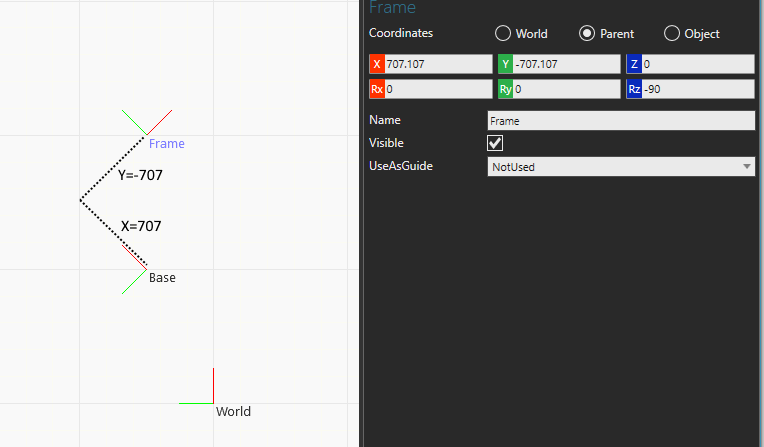For transformation matrix from Base to Frame the location and orientation of World frame is unknown so you cannot expect its values to use World as reference in any way. So in your case Measure tool returns offsets in world coordinates but your transformation matrix uses Base as reference so there is a mismatch in position values.

-k